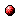# Fuzzy partition optimization for approximate fuzzy Q-iteration

Reference:
L. Busoniu, D. Ernst, B. De Schutter, and R. Babuska, "Fuzzy partition optimization for approximate fuzzy Q-iteration," Proceedings of the 17th IFAC World Congress, Seoul, Korea, pp. 5629-5634, July 2008.

Abstract:
Reinforcement Learning (RL) is a widely used learning paradigm for adaptive agents. Because exact RL can only be applied to very simple problems, approximate algorithms are usually necessary in practice. Many algorithms for approximate RL rely on basis-function representations of the value function (or of the Q-function). Designing a good set of basis functions without any prior knowledge of the value function (or of the Q-function) can be a difficult task. In this paper, we propose instead a technique to optimize the shape of a constant number of basis functions for the approximate, fuzzy Q-iteration algorithm. In contrast to other approaches to adapt basis functions for RL, our optimization criterion measures the actual performance of the computed policies in the task, using simulation from a representative set of initial states. A complete algorithm, using cross-entropy optimization of triangular fuzzy membership functions, is given and applied to the car-on-the-hill example.Online version of the paperCorresponding technical report: pdf file (225 KB)
Note: More information on the pdf file format mentioned above can be found here.

Bibtex entry:

@inproceedings{BusErn:07-035,
author={L. Bu{\c{s}}oniu and D. Ernst and B. {D}e Schutter and R. Babu{\v{s}}ka},
title={Fuzzy partition optimization for approximate fuzzy {Q}-iteration},
booktitle={Proceedings of the 17th IFAC World Congress},